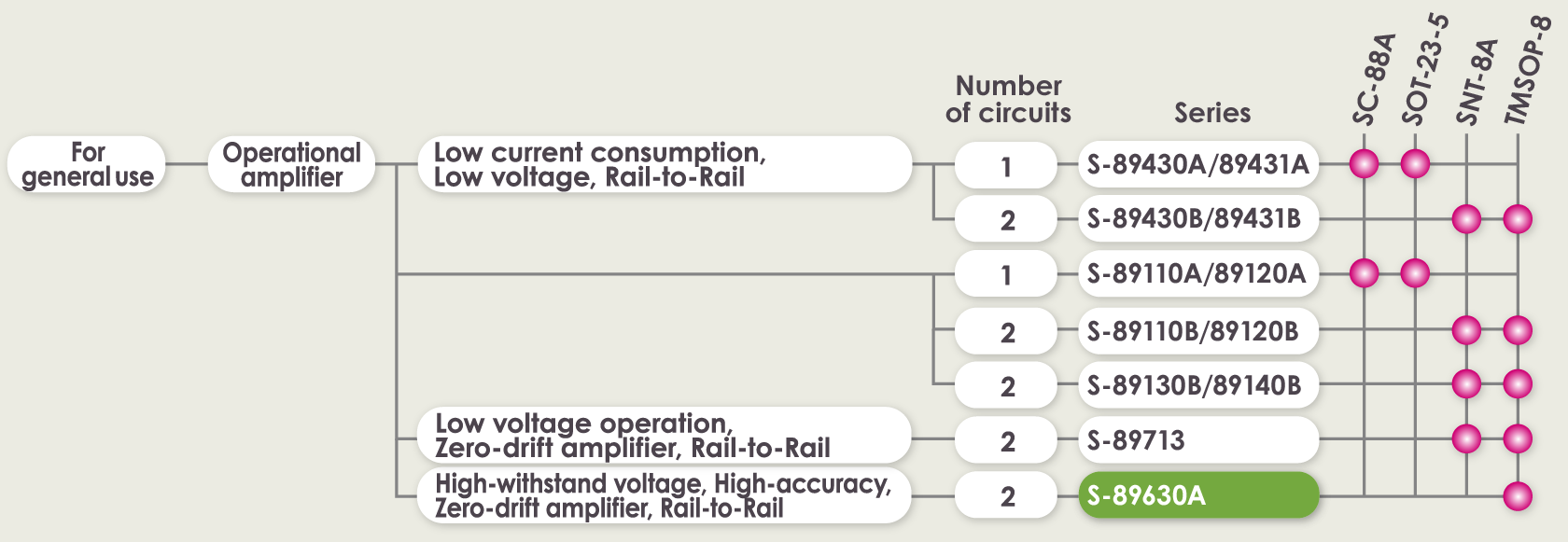Amplifiers

# What is an Operational Amplifier?

## 1. What is an Operational Amplifier (Op-amp)?

An operational amplifier is an integrated circuit that can amplify weak electric signals.
An operational amplifier has two input pins and one output pin. Its basic role is to amplify and output the voltage difference between the two input pins.

## 2. What an Operational Amplifier Can Do

An operational amplifier is not used alone but is designed to be connected to other circuits to perform a great variety of operations. This article provides some typical examples of usage of circuits with operational amplifiers.

#### ●Enables substantial amplification of an input signal

When an operational amplifier is combined with an amplification circuit, it can amplify weak signals to strong signals.It behaves like a megaphone where the input signal is a person’s voice and the megaphone is the operational amplifier circuit.For example, such a circuit can be used to amplify minute sensor signals.Processing of sensor signals can be further improved by inputting the amplified signal to a microcontroller* unit (MCU).
* Microcontroller… A compact computer for controlling electronic devices. As the brain of electronic devices, MCUs operate according to input signals.

#### ●Enables elimination of noise from an input signal

By operating as a filter of input signals, the operational amplifier circuit is able to extract the signal with the target frequency.For example, when an operational amplifier circuit is used for voice recognition or in a voice recorder, it can extract frequencies close to the targeted sound while shutting out all other frequencies as noise.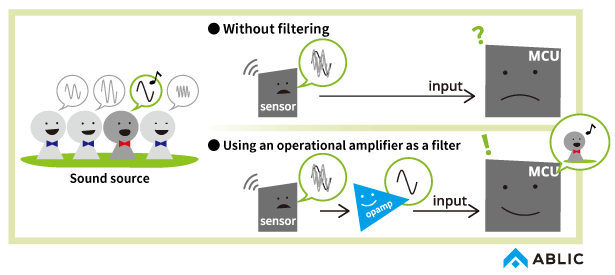An operational amplifier circuit can be tweaked to perform a broad range of functions such as arithmetical operations or signal synthesis.

## 3. Operational Amplifier Applications

As noted above, an operational amplifier is almost never used alone. By connecting resistors or capacitors, you can configure a circuit capable of the signal amplification, filtering or arithmetic circuit operations described in “2. What an Operational Amplifier Can Do”.

#### (1)Internal operational amplifier operations

The following describes the operations performed by the operational amplifier in the circuit. Let’s see how an operational amplifier behaves in an amplifier circuit, taking the example of a non-inverting amplifier circuit.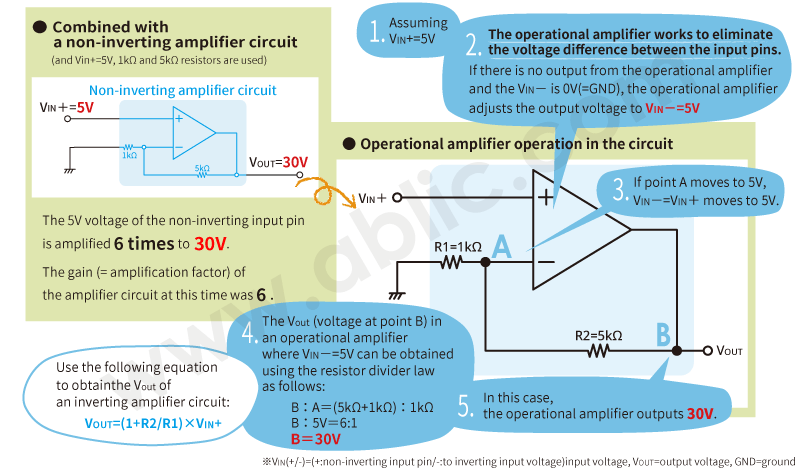In addition to this simple calculation, the operational amplifier’s performance must also be considered when configuring a circuit. This point is described later in this section under “4. Selecting an Operational Amplifier and Explanation of Terms.

#### (2)Circuit examples

We describe here some typical operational amplifier applications.

[Non-inverting amplifier circuit]

As explained in (1), this also is a circuit for amplifying and outputting input signals.

• VOUT=(1+R2/R1)×VIN

[Inverting amplifier circuit]

An inverting amplifier circuit is indicated by a minus sign. If the VIN voltage increases, the VOUT voltage decreases.

• VOUT=-R2/R1×VIN

[Voltage follower circuit]

This is a non-inverting amplifier circuit where R2 is short-circuited (R2=0Ω) and R1 is open (R1=infinity). Since VOUT=(1+R2/R1)×VIN= (1+0Ω/∞)×VIN=VIN, the output is the same voltage as the input signal. A voltage follower is used as a buffer circuit to convert the impedance or to separate circuits.

• VOUT=VIN

[Differential amplifier circuit]

This is a circuit for amplifying and outputting the difference between two input signals.

• VOUT=R2/R1×(VIN2-VIN1)

## 4. Selecting an Operational Amplifier and Explanation of Terms

Here, we will use the ABLIC operational amplifier S-89630A as an example of what items to check in selecting an operational amplifier and explaining operational amplifier attributes.

#### (1) Check the operating voltage.

[Operating power supply voltage range]
This is the range of the operable power supply voltage at the VDD pin.Check that the power supply voltage is within the range of the operational amplifier operating voltage range.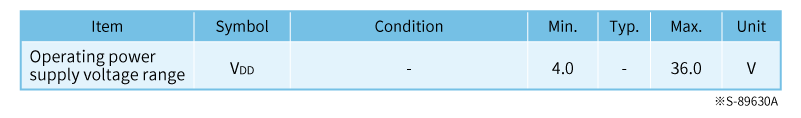[Common-mode input voltage range]
The range of voltage of a signal that may be applied to the input pins. The operational amplifier will work as long as the input signal is within this range.An operational amplifier whose common-mode input voltage range covers VSS – VDD is called a “Rail-to-Rail input operational amplifier”; that is, an operational amplifier with an excellent input signal voltage range.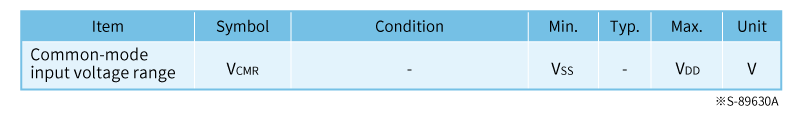#### (2) Check the input signal frequency.

[Gain bandwidth product]
This specifies the maximum frequency that an operational amplifier can amplify a signal to. The maximum frequency varies with the factor (gain) you use to amplify a signal. At a gain of one (=0dB), the signal can be amplified to maximum frequency, the so called gain bandwidth product.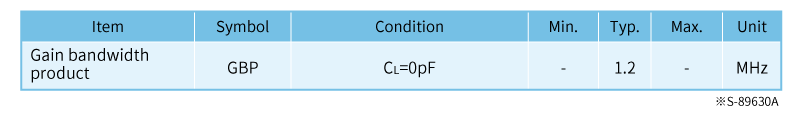The graph on the right indicates that at a gain of one (=0dB), the maximum frequency that amplification allows using the S-89630A is 1.2MHz and that at a gain of 10 (=20dB), the maximum frequency is 120kHz.Make sure that the maximum frequency you want to amplify to is within the range of the factor by which you want to amplify.

#### (3) Check the current consumption.

[Current consumption]
This indicates the current value drawn from the VDD pin. The lower this value is, the more you can reduce the power of the system. Normally, an operational amplifier with low current consumption tends to also have low frequency of gain bandwidth.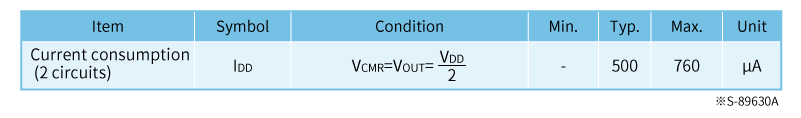#### (4) Check the signal amplification accuracy.

[Input offset voltage]
The input offset voltage is a conversion of the error voltage generated at the output to an input value when the input voltage is 0V. It is an essential attribute affecting the amplification accuracy of operational amplifiers. Generally, when the voltage amplitude of the input signal is on the order of mV, an input offset voltage on the order of μV is required. This makes it necessary to select a “zero drift operational amplifier” to handle such tiny offset voltages.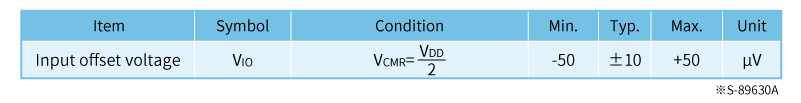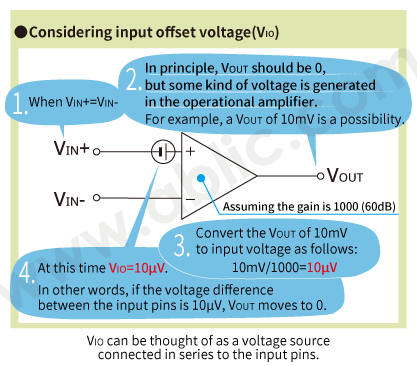## 5. What is a Zero Drift Amplifier?

A zero drift amplifier is an operational amplifier that minimizes input offset voltage and input offset voltage drift (≒0).Selecting a zero-drift operational amplifier is a highly effective solution for applications that demand high-accuracy signal amplification.

Describes zero drift amplifier principle!
What is a Zero Drift Amplifier?

## 6. ABLIC’s Operational Amplifiers

ABLIC’s operational amplifiers feature low current consumption and low-voltage drive and are available in packages as small as 2.0×2.1 mm.
In addition to products for general use that can be used in a wide range of applications including industrial equipment, we also have precisely developed a lineup of automotive products that are AEC-Q100 qualified and PPAP capable. When looking for an operational amplifier, consider ABLIC’s operational amplifiers.

##### Operational amplifiers for general use